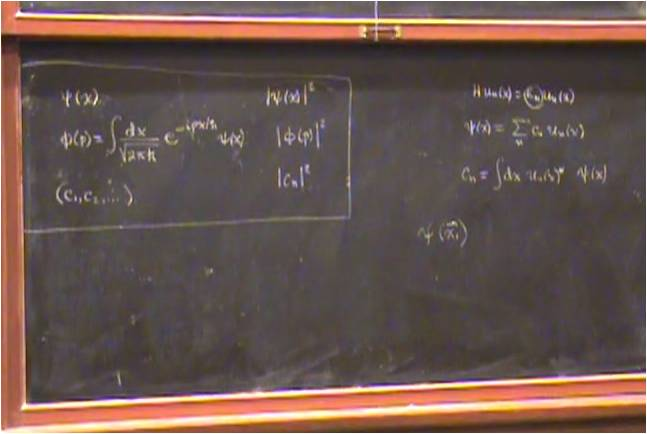# InfoCoBuild

## Physics 221A: Quantum Mechanics

Physics 221A: Quantum Mechanics (Fall 2010, UC Berkeley). Instructor: Professor Robert Littlejohn. This course deals with topics in quantum mechanics: basic assumptions of quantum mechanics; quantum theory of measurement; matrix mechanics; Schroedinger theory; symmetry and invariance principles; theory of angular momentum; stationary state problems; variational principles; time independent perturbation theory; time dependent perturbation theory; theory of scattering. The textbook for this course is Modern Quantum Mechanics by J.J. Sakurai, Revised Edition (Addison-Wesley, New York, 1994).Lecture 01 - The Mathematical Formalism of Quantum Mechanics Lecture 02 - The Mathematical Formalism of Quantum Mechanics (cont.) Lecture 03 - The Mathematical Formalism (cont.), The Postulates of Quantum Mechanics Lecture 04 - The Postulates of Quantum Mechanics (cont.), The Density Operator Lecture 05 - The Density Operator (cont.), Spatial Degrees of Freedom Lecture 06 - Spatial Degrees of Freedom (cont.) Lecture 07 - Time Evolution in Quantum Mechanics Lecture 08 - Time Evolution in Quantum Mechanics (cont.), Topics in One-Dimensional Wave Mechanics, The WKB Method Lecture 09 - The WKB Method (cont.) Lecture 10 - The WKB Method (cont.) Lecture 11 - The WKB Method (cont.), Harmonic Oscillators and Coherent States Lecture 12 - Harmonic Oscillators and Coherent States (cont.) Lecture 13 - Harmonic Oscillators and Coherent States (cont.), The Propagator and the Path Integral Lecture 14 - The Propagator and the Path Integral (cont.) Lecture 15 - The Propagator and the Path Integral (cont.) Lecture 16 - The Propagator and the Path Integral (cont.), Charged Particles in Magnetic Fields Lecture 17 - Charged Particles in Magnetic Fields (cont.) Lecture 18 - Charged Particles in Magnetic Fields (cont.), Rotations in Ordinary Space Lecture 19 - Rotations in Ordinary Space (cont.), Rotations in Quantum Mechanics, and Rotations of Spin 1/2 Systems Lecture 20 - Rotations in Quantum Mechanics, and Rotations of Spin 1/2 Systems Lecture 21 - Rotations in Quantum Mechanics, and Rotations of Spin 1/2 Systems (cont.), Representations of the Angular Momentum Operators and Rotations Lecture 22 - Representations of the Angular Momentum Operators and Rotations, Spins in Magnetic Fields Lecture 23 - Spins in Magnetic Fields (cont.) Lecture 24 - Spins in Magnetic Fields (cont.), Orbital Angular Momentum and Spherical Harmonics Lecture 25 - Orbital Angular Momentum and Spherical Harmonics (cont.), Central Force Motion Lecture 26 - Central Force Motion (cont.) Lecture 27 - Central Force Motion (cont.) Lecture 28 - Coupling Spatial and Spin Degrees of Freedom, Coupling of Angular Momenta Lecture 29 - Coupling of Angular Momenta (cont.) Lecture 30 - Irreducible Tensor Operators and the Wigner-Eckart Theorem Lecture 31 - Irreducible Tensor Operators and the Wigner-Eckart Theorem (cont.) Lecture 32 - Irreducible Tensor Operators and the Wigner-Eckart Theorem (cont.), Parity Lecture 33 - Parity (cont.), Time Reversal Lecture 34 - Time Reversal (cont.) Lecture 35 - Time Reversal (cont.) Lecture 36 - Bound-State Perturbation Theory Lecture 37 - The Stark Effect in Hydrogen and Alkali Atoms Lecture 38 - The Stark Effect in Hydrogen and Alkali Atoms, Fine Structure in Hydrogen and Alkali Atoms Lecture 39 - Fine Structure in Hydrogen and Alkali Atoms, The Zeeman Effect in Hydrogen and Alkali Atoms Lecture 40 - The Zeeman Effect in Hydrogen and Alkali Atoms (cont.) Lecture 41 - Deuteron

 Related Links Physics 221A Quantum Mechanics, Fall 2010 Instructor: Robert Littlejohn. Lecture Notes. Homework Assignments. Homework Solutions. Recommended Text: Modern Quantum Mechanics by J.J. Sakurai, Revised Edition.